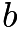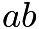# Nov 2016

I will post seven new problems every weekend, one for each day of the week. The idea is that you work on your own during the week and I will post answers the following Saturday.  For those of you who practiced with me last year, the process is similar.

For instructions on submitting weekly answers, click on the “Logistics” tab.
For previously posted problems, click on the “Archive” tab.
For previous prizes, click on the “News” tab.

Week of Nov 6 – Nov 12:  This is the last week before ACM 8 takes place on Nov 15.  We will do another timed practice this week.  Again, 40 minutes, 1 sitting, no calculator, no team work, no web help, and also no penalty for GUESSING. Like last week, I will also ask you to submit your solutions, not just the multiple choice answers, to three of the harder problems at the end of the test. This will give you a chance to work on the harder problems if you don’t get to finish the entire test in 40 minutes.  The best solution(s) will be posted and acknowledged.

Click Googleform to submit solutions to the 3 written problems by Nov 12.

Nov 6: AMC8 test 2011, https://artofproblemsolving.com/wiki/index.php?title=2011_AMC_8_Problems.  Please take the test before looking at the three problems below.

Nov 7-8:  (Problem 22) What is the tens digit of$7^{2011}$?

Nov 9-10:  (Problem 23) How many 4-digit positive integers have four different digits, where the leading digit is not zero, the integer is a multiple of 5, and 5 is the largest digit?

Nov 11-12: (Problem 24) In how many ways can 10001 be written as the sum of two primes?

Week of Oct 30 – Nov 5: We will do another timed practice this week.  Again, 40 minutes, 1 sitting, no calculator, no team work, no web help, and also no penalty for GUESSING. Like last week, I will also ask you to submit your solutions, not just the multiple choice answers, to three of the harder problems at the end of the test. This will give you a chance to work on the harder problems if you don’t get to finish the entire test in 40 minutes.  The best solution(s) will be posted and acknowledged.

Click Googleform to submit solutions to the 3 written problems by Nov 5.  See solutions: https://artofproblemsolving.com/wiki/index.php?title=2011_AMC_8_Answer_Key

Oct 30: AMC8 test 2012, http://artofproblemsolving.com/wiki/index.php?title=2012_AMC_8_Problems.  Please take the test before looking at the three problems below.

Oct 31- Nov 1:  (Problem 18)  What is the smallest positive integer that is neither prime nor square and that has no prime factor less than 50?

[Om’s solution]  The smallest prime number greater than 50 is 53. This can’t be the answer though, because it is prime. This means you have to multiply it by a number, and that number will have to be a prime number greater than 50. It can’t be 53 though, because then the answer would be a square. So you multiply 53 by the next largest prime, 59, to get 3127.

Nov 2 – 3:  (Problem 23) An equilateral triangle and a regular hexagon have equal perimeters. If the area of the triangle is 4, what is the area of the hexagon?

[Stephen’s solution] Let’s call the side length of the triangle s. Since the triangle and and the hexagon have the same perimeter the side length of the hexagon is s/2. Then we can divide the hexagon into 6 equilateral triangles. Those triangles each has an area quarter to the big triangle. 4*(1/4)=1. 1*6=6

Nov 4-5:  (Problem 25) A square with area 4 is inscribed in a square with area 5, with one vertex of the smaller square on each side of the larger square. A vertex of the smaller square divides a side of the larger square into two segments, one of length$a$, and the other of length$b$. What is the value of$ab$?$[asy] draw((0,2)--(2,2)--(2,0)--(0,0)--cycle); draw((0,0.3)--(0.3,2)--(2,1.7)--(1.7,0)--cycle); label($

[Stephen’s solution]
a^2+b^2=4 (due to pythagorean)
a+b=sqrt(5) (side length of square)
(a+b)^2-2ab=4 (re-write first equation)
5-2ab=4 (apply second equation)
2ab=1 (simplify)
ab=1/2 (simplify)

[Om’s solution] We know that one square has an area of 4, and the larger one has an area of 5. Subtracting 4 from 5 shows that the area of the 4 triangles(which are the areas inside the large square and outside the small square) is 1. This means that the area of one triangle is 1/4, or 0.25. A is the width and b is the height, so A*B*1/2 is the area, which is 1/4. You multiply by two to get A*B, which is 1/2. We could try to find A and B individually, but the problem asks to find A*B, which we have. The answer is 1/2.